# Extracting and visualizing tidy draws from brms models

## Introduction

This vignette describes how to use the `tidybayes` and `ggdist` packages to extract and visualize tidy data frames of draws from posterior distributions of model variables, fits, and predictions from `brms::brm`. For a more general introduction to `tidybayes` and its use on general-purpose Bayesian modeling languages (like Stan and JAGS), see `vignette("tidybayes")`.

## Setup

The following libraries are required to run this vignette:

``````library(magrittr)
library(dplyr)
library(purrr)
library(forcats)
library(tidyr)
library(modelr)
library(ggdist)
library(tidybayes)
library(ggplot2)
library(cowplot)
library(rstan)
library(brms)
library(ggrepel)
library(RColorBrewer)
library(gganimate)

theme_set(theme_tidybayes() + panel_border())``````

These options help Stan run faster:

``````rstan_options(auto_write = TRUE)
options(mc.cores = parallel::detectCores())``````

## Example dataset

To demonstrate `tidybayes`, we will use a simple dataset with 10 observations from 5 conditions each:

``````set.seed(5)
n = 10
n_condition = 5
ABC =
tibble(
condition = rep(c("A","B","C","D","E"), n),
response = rnorm(n * 5, c(0,1,2,1,-1), 0.5)
)``````

A snapshot of the data looks like this:

``head(ABC, 10)``
condition response
A -0.4204277
B 1.6921797
C 1.3722541
D 1.0350714
E -0.1442796
A -0.3014540
B 0.7639168
C 1.6823143
D 0.8571132
E -0.9309459

This is a typical tidy format data frame: one observation per row. Graphically:

``````ABC %>%
ggplot(aes(y = condition, x = response)) +
geom_point()``````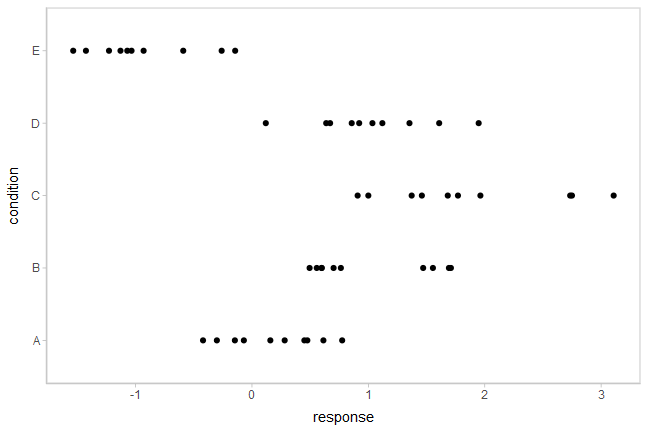## Model

Let’s fit a hierarchical model with shrinkage towards a global mean:

``````m = brm(
response ~ (1|condition),
data = ABC,
prior = c(
prior(normal(0, 1), class = Intercept),
prior(student_t(3, 0, 1), class = sd),
prior(student_t(3, 0, 1), class = sigma)
),

file = "models/tidy-brms_m.rds" # cache model (can be removed)
)``````

The results look like this:

``m``
``````##  Family: gaussian
##   Links: mu = identity; sigma = identity
## Formula: response ~ (1 | condition)
##    Data: ABC (Number of observations: 50)
## Samples: 4 chains, each with iter = 2000; warmup = 1000; thin = 1;
##          total post-warmup samples = 4000
##
## Group-Level Effects:
## ~condition (Number of levels: 5)
##               Estimate Est.Error l-95% CI u-95% CI Rhat Bulk_ESS Tail_ESS
## sd(Intercept)     1.15      0.42     0.61     2.23 1.00      991     1353
##
## Population-Level Effects:
##           Estimate Est.Error l-95% CI u-95% CI Rhat Bulk_ESS Tail_ESS
## Intercept     0.51      0.47    -0.46     1.40 1.00     1001     1198
##
## Family Specific Parameters:
##       Estimate Est.Error l-95% CI u-95% CI Rhat Bulk_ESS Tail_ESS
## sigma     0.56      0.06     0.45     0.70 1.00     1538     1309
##
## Samples were drawn using sampling(NUTS). For each parameter, Bulk_ESS
## and Tail_ESS are effective sample size measures, and Rhat is the potential
## scale reduction factor on split chains (at convergence, Rhat = 1).``````

## Extracting draws from a fit in tidy-format using `spread_draws`

Now that we have our results, the fun begins: getting the draws out in a tidy format! First, we’ll use the `get_variables()` function to get a list of raw model variable names so that we know what variables we can extract from the model:

``get_variables(m)``
``````##   "b_Intercept"              "sd_condition__Intercept"  "sigma"                    "r_condition[A,Intercept]"
##   "r_condition[B,Intercept]" "r_condition[C,Intercept]" "r_condition[D,Intercept]" "r_condition[E,Intercept]"
##   "lp__"                     "accept_stat__"            "stepsize__"               "treedepth__"
##  "n_leapfrog__"             "divergent__"              "energy__"``````

Here, `b_Intercept` is the global mean, and the `r_condition[]` variables are offsets from that mean for each condition. Given these variables:

• `r_condition[A,Intercept]`
• `r_condition[B,Intercept]`
• `r_condition[C,Intercept]`
• `r_condition[D,Intercept]`
• `r_condition[E,Intercept]`

We might want a data frame where each row is a draw from either `r_condition[A,Intercept]`, `r_condition[B,Intercept]`, `...[C,...]`, `...[D,...]`, or `...[E,...]`, and where we have columns indexing which chain/iteration/draw the row came from and which condition (`A` to `E`) it is for. That would allow us to easily compute quantities grouped by condition, or generate plots by condition using ggplot, or even merge draws with the original data to plot data and posteriors simultaneously.

The workhorse of `tidybayes` is the `spread_draws()` function, which does this extraction for us. It includes a simple specification format that we can use to extract variables and their indices into tidy-format data frames.

### Gathering variable indices into a separate column in a tidy format data frame

Given a variable in the model like this:

`r_condition[D,Intercept]`

We can provide `spread_draws()` with a column specification like this:

`r_condition[condition,term]`

Where `condition` corresponds to `D` and `term` corresponds to `Intercept`. There is nothing too magical about what `spread_draws()` does with this specification: under the hood, it splits the variable indices by commas and spaces (you can split by other characters by changing the `sep` argument). It lets you assign columns to the resulting indices in order. So `r_condition[D,Intercept]` has indices `D` and `Intercept`, and `spread_draws()` lets us extract these indices as columns in the resulting tidy data frame of draws from `r_condition`:

``````m %>%
condition term r_condition .chain .iteration .draw
A Intercept -0.4584587 1 1 1
A Intercept -0.8497705 1 2 2
A Intercept -0.1291771 1 3 3
A Intercept -0.0975620 1 4 4
A Intercept 0.0627511 1 5 5
A Intercept -0.5170882 1 6 6
A Intercept -0.4955497 1 7 7
A Intercept -1.0082885 1 8 8
A Intercept -0.3525897 1 9 9
A Intercept -0.3523500 1 10 10

We can choose whatever names we want for the index columns; e.g.:

``````m %>%
c t r_condition .chain .iteration .draw
A Intercept -0.4584587 1 1 1
A Intercept -0.8497705 1 2 2
A Intercept -0.1291771 1 3 3
A Intercept -0.0975620 1 4 4
A Intercept 0.0627511 1 5 5
A Intercept -0.5170882 1 6 6
A Intercept -0.4955497 1 7 7
A Intercept -1.0082885 1 8 8
A Intercept -0.3525897 1 9 9
A Intercept -0.3523500 1 10 10

But the more descriptive and less cryptic names from the previous example are probably preferable.

In this particular model, there is only one term (`Intercept`), thus we could omit that index altogether to just get each `condition` and the value of `r_condition` for that condition:

``````m %>%
condition r_condition .chain .iteration .draw
A -0.4584587 1 1 1
A -0.8497705 1 2 2
A -0.1291771 1 3 3
A -0.0975620 1 4 4
A 0.0627511 1 5 5
A -0.5170882 1 6 6
A -0.4955497 1 7 7
A -1.0082885 1 8 8
A -0.3525897 1 9 9
A -0.3523500 1 10 10

Note: If you have used `spread_draws()` with a raw sample from Stan or JAGS, you may be used to using `recover_types` before `spread_draws()` to get index column values back (e.g. if the index was a factor). This is not necessary when using `spread_draws()` on `rstanarm` models, because those models already contain that information in their variable names. For more on `recover_types`, see `vignette("tidybayes")`.

## Point summaries and intervals

### With simple model variables

`tidybayes` provides a family of functions for generating point summaries and intervals from draws in a tidy format. These functions follow the naming scheme `[median|mean|mode]_[qi|hdi]`, for example, `median_qi()`, `mean_qi()`, `mode_hdi()`, and so on. The first name (before the `_`) indicates the type of point summary, and the second name indicates the type of interval. `qi` yields a quantile interval (a.k.a. equi-tailed interval, central interval, or percentile interval) and `hdi` yields a highest (posterior) density interval. Custom point summary or interval functions can also be applied using the `point_interval()` function.

For example, we might extract the draws corresponding to posterior distributions of the overall mean and standard deviation of observations:

``````m %>%
.chain .iteration .draw b_Intercept sigma
1 1 1 0.6202164 0.5278706
1 2 2 0.9549294 0.5100162
1 3 3 0.3679654 0.5702053
1 4 4 0.4164658 0.6413618
1 5 5 0.0676259 0.5562091
1 6 6 0.7080753 0.5485105
1 7 7 0.7753490 0.5682802
1 8 8 0.9808815 0.5251010
1 9 9 0.6134809 0.4911794
1 10 10 0.5887487 0.5070235

Like with `r_condition[condition,term]`, this gives us a tidy data frame. If we want the median and 95% quantile interval of the variables, we can apply `median_qi()`:

``````m %>%
median_qi(b_Intercept, sigma)``````
b_Intercept b_Intercept.lower b_Intercept.upper sigma sigma.lower sigma.upper .width .point .interval
0.5277919 -0.4646531 1.396154 0.5560855 0.4540873 0.698126 0.95 median qi

We can specify the columns we want to get medians and intervals from, as above, or if we omit the list of columns, `median_qi()` will use every column that is not a grouping column or a special column (like `.chain`, `.iteration`, or `.draw`). Thus in the above example, `b_Intercept` and `sigma` are redundant arguments to `median_qi()` because they are also the only columns we gathered from the model. So we can simplify this to:

``````m %>%
median_qi()``````
b_Intercept b_Intercept.lower b_Intercept.upper sigma sigma.lower sigma.upper .width .point .interval
0.5277919 -0.4646531 1.396154 0.5560855 0.4540873 0.698126 0.95 median qi

If you would rather have a long-format list of intervals, use `gather_draws()` instead:

``````m %>%
gather_draws(b_Intercept, sigma) %>%
median_qi()``````
.variable .value .lower .upper .width .point .interval
b_Intercept 0.5277919 -0.4646531 1.396154 0.95 median qi
sigma 0.5560855 0.4540873 0.698126 0.95 median qi

For more on `gather_draws()`, see `vignette("tidybayes")`.

### With indexed model variables

When we have a model variable with one or more indices, such as `r_condition`, we can apply `median_qi()` (or other functions in the `point_interval()` family) as we did before:

``````m %>%
median_qi()``````
condition r_condition .lower .upper .width .point .interval
A -0.3388408 -1.2387503 0.7064627 0.95 median qi
B 0.4661538 -0.4377360 1.5471919 0.95 median qi
C 1.2938263 0.4002230 2.3704153 0.95 median qi
D 0.4853882 -0.4192433 1.5141353 0.95 median qi
E -1.4074598 -2.3590914 -0.3934355 0.95 median qi

How did `median_qi()` know what to aggregate? Data frames returned by `spread_draws()` are automatically grouped by all index variables you pass to it; in this case, that means `spread_draws()` groups its results by `condition`. `median_qi()` respects those groups, and calculates the point summaries and intervals within all groups. Then, because no columns were passed to `median_qi()`, it acts on the only non-special (`.`-prefixed) and non-group column, `r_condition`. So the above shortened syntax is equivalent to this more verbose call:

``````m %>%
group_by(condition) %>%   # this line not necessary (done by spread_draws)
median_qi(r_condition)      # b is not necessary (it is the only non-group column)``````
condition r_condition .lower .upper .width .point .interval
A -0.3388408 -1.2387503 0.7064627 0.95 median qi
B 0.4661538 -0.4377360 1.5471919 0.95 median qi
C 1.2938263 0.4002230 2.3704153 0.95 median qi
D 0.4853882 -0.4192433 1.5141353 0.95 median qi
E -1.4074598 -2.3590914 -0.3934355 0.95 median qi

## Combining variables with different indices in a single tidy format data frame

`spread_draws()` and `gather_draws()` support extracting variables that have different indices into the same data frame. Indices with the same name are automatically matched up, and values are duplicated as necessary to produce one row per all combination of levels of all indices. For example, we might want to calculate the mean within each condition (call this `condition_mean`). In this model, that mean is the intercept (`b_Intercept`) plus the effect for a given condition (`r_condition`).

We can gather draws from `b_Intercept` and `r_condition` together in a single data frame:

``````m %>%
.chain .iteration .draw b_Intercept condition r_condition
1 1 1 0.6202164 A -0.4584587
1 1 1 0.6202164 B 0.5522848
1 1 1 0.6202164 C 1.5236969
1 1 1 0.6202164 D 0.2207564
1 1 1 0.6202164 E -1.3820600
1 2 2 0.9549294 A -0.8497705
1 2 2 0.9549294 B -0.1369764
1 2 2 0.9549294 C 0.8056390
1 2 2 0.9549294 D 0.1813015
1 2 2 0.9549294 E -1.9581073

Within each draw, `b_Intercept` is repeated as necessary to correspond to every index of `r_condition`. Thus, the `mutate` function from dplyr can be used to find their sum, `condition_mean` (which is the mean for each condition):

``````m %>%
mutate(condition_mean = b_Intercept + r_condition) %>%
median_qi(condition_mean)``````
condition condition_mean .lower .upper .width .point .interval
A 0.1902109 -0.1459784 0.5289075 0.95 median qi
B 0.9987517 0.6413898 1.3512293 0.95 median qi
C 1.8323895 1.4717317 2.1825785 0.95 median qi
D 1.0102834 0.6568990 1.3549014 0.95 median qi
E -0.8914270 -1.2497696 -0.5332862 0.95 median qi

`median_qi()` uses tidy evaluation (see `vignette("tidy-evaluation", package = "rlang")`), so it can take column expressions, not just column names. Thus, we can simplify the above example by moving the calculation of `condition_mean` from `mutate` into `median_qi()`:

``````m %>%
median_qi(condition_mean = b_Intercept + r_condition)``````
condition condition_mean .lower .upper .width .point .interval
A 0.1902109 -0.1459784 0.5289075 0.95 median qi
B 0.9987517 0.6413898 1.3512293 0.95 median qi
C 1.8323895 1.4717317 2.1825785 0.95 median qi
D 1.0102834 0.6568990 1.3549014 0.95 median qi
E -0.8914270 -1.2497696 -0.5332862 0.95 median qi

## Plotting point summaries and intervals

Plotting point summaries and with one interval is straightforward using `ggdist::geom_pointinterval()`:

``````m %>%
median_qi(condition_mean = b_Intercept + r_condition) %>%
ggplot(aes(y = condition, x = condition_mean, xmin = .lower, xmax = .upper)) +
geom_pointinterval()``````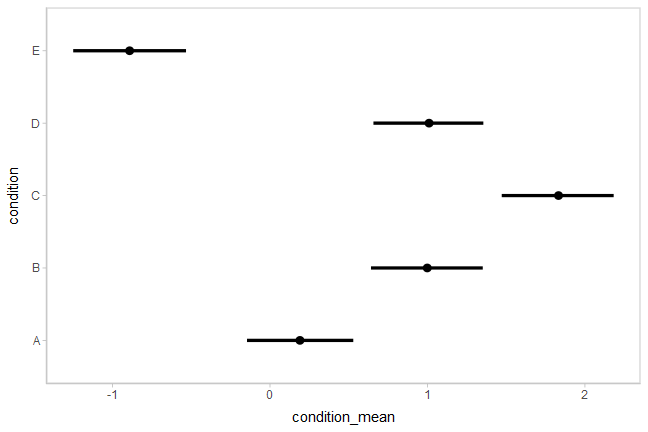## Intervals with multiple probability levels

`median_qi()` and its sister functions can also produce an arbitrary number of probability intervals by setting the `.width =` argument:

``````m %>%
median_qi(condition_mean = b_Intercept + r_condition, .width = c(.95, .8, .5))``````
condition condition_mean .lower .upper .width .point .interval
A 0.1902109 -0.1459784 0.5289075 0.95 median qi
B 0.9987517 0.6413898 1.3512293 0.95 median qi
C 1.8323895 1.4717317 2.1825785 0.95 median qi
D 1.0102834 0.6568990 1.3549014 0.95 median qi
E -0.8914270 -1.2497696 -0.5332862 0.95 median qi
A 0.1902109 -0.0336112 0.4157260 0.80 median qi
B 0.9987517 0.7670282 1.2268020 0.80 median qi
C 1.8323895 1.5960726 2.0533486 0.80 median qi
D 1.0102834 0.7862752 1.2367393 0.80 median qi
E -0.8914270 -1.1249791 -0.6483463 0.80 median qi
A 0.1902109 0.0708217 0.3127687 0.50 median qi
B 0.9987517 0.8760232 1.1155393 0.50 median qi
C 1.8323895 1.7050903 1.9524725 0.50 median qi
D 1.0102834 0.8922209 1.1278207 0.50 median qi
E -0.8914270 -1.0133196 -0.7681952 0.50 median qi

The results are in a tidy format: one row per group and uncertainty interval width (`.width`). This facilitates plotting. For example, assigning `-.width` to the `size` aesthetic will show all intervals, making thicker lines correspond to smaller intervals. The `ggdist::geom_pointinterval()` geom automatically sets the `size` aesthetic appropriately based on the `.width` column in the data to produce plots of points with multiple probability levels:

``````m %>%
median_qi(condition_mean = b_Intercept + r_condition, .width = c(.95, .66)) %>%
ggplot(aes(y = condition, x = condition_mean, xmin = .lower, xmax = .upper)) +
geom_pointinterval() ``````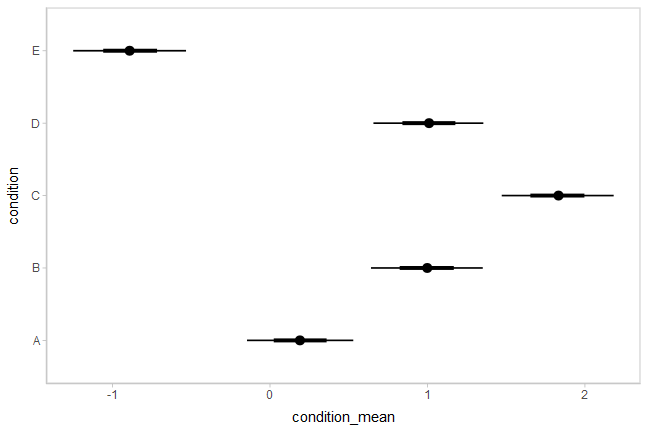## Intervals with densities

To see the density along with the intervals, we can use `ggdist::stat_eye()` (“eye plots”, which combine intervals with violin plots), or `ggdist::stat_halfeye()` (interval + density plots):

``````m %>%
mutate(condition_mean = b_Intercept + r_condition) %>%
ggplot(aes(y = condition, x = condition_mean)) +
stat_halfeye()``````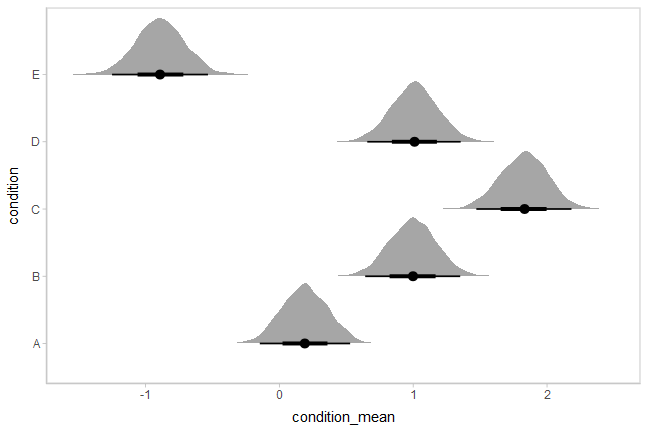Or say you want to annotate portions of the densities in color; the `fill` aesthetic can vary within a slab in all geoms and stats in the `ggdist::geom_slabinterval()` family, including `ggdist::stat_halfeye()`. For example, if you want to annotate a domain-specific region of practical equivalence (ROPE), you could do something like this:

``````m %>%
mutate(condition_mean = b_Intercept + r_condition) %>%
ggplot(aes(y = condition, x = condition_mean, fill = stat(abs(x) < .8))) +
stat_halfeye() +
geom_vline(xintercept = c(-.8, .8), linetype = "dashed") +
scale_fill_manual(values = c("gray80", "skyblue"))``````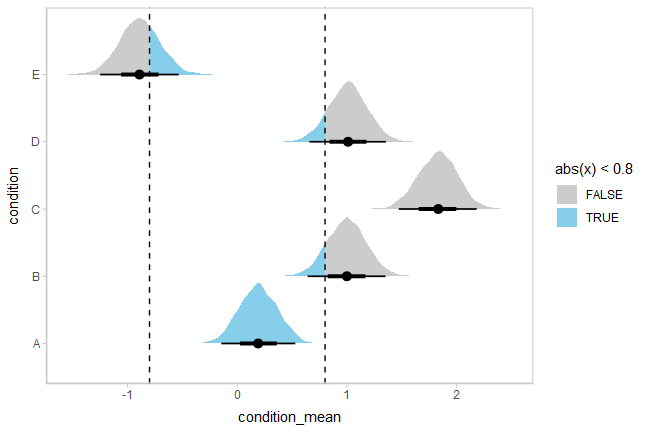## Other visualizations of distributions: `stat_slabinterval`

There are a variety of additional stats for visualizing distributions in the `ggdist::geom_slabinterval()` family of stats and geoms: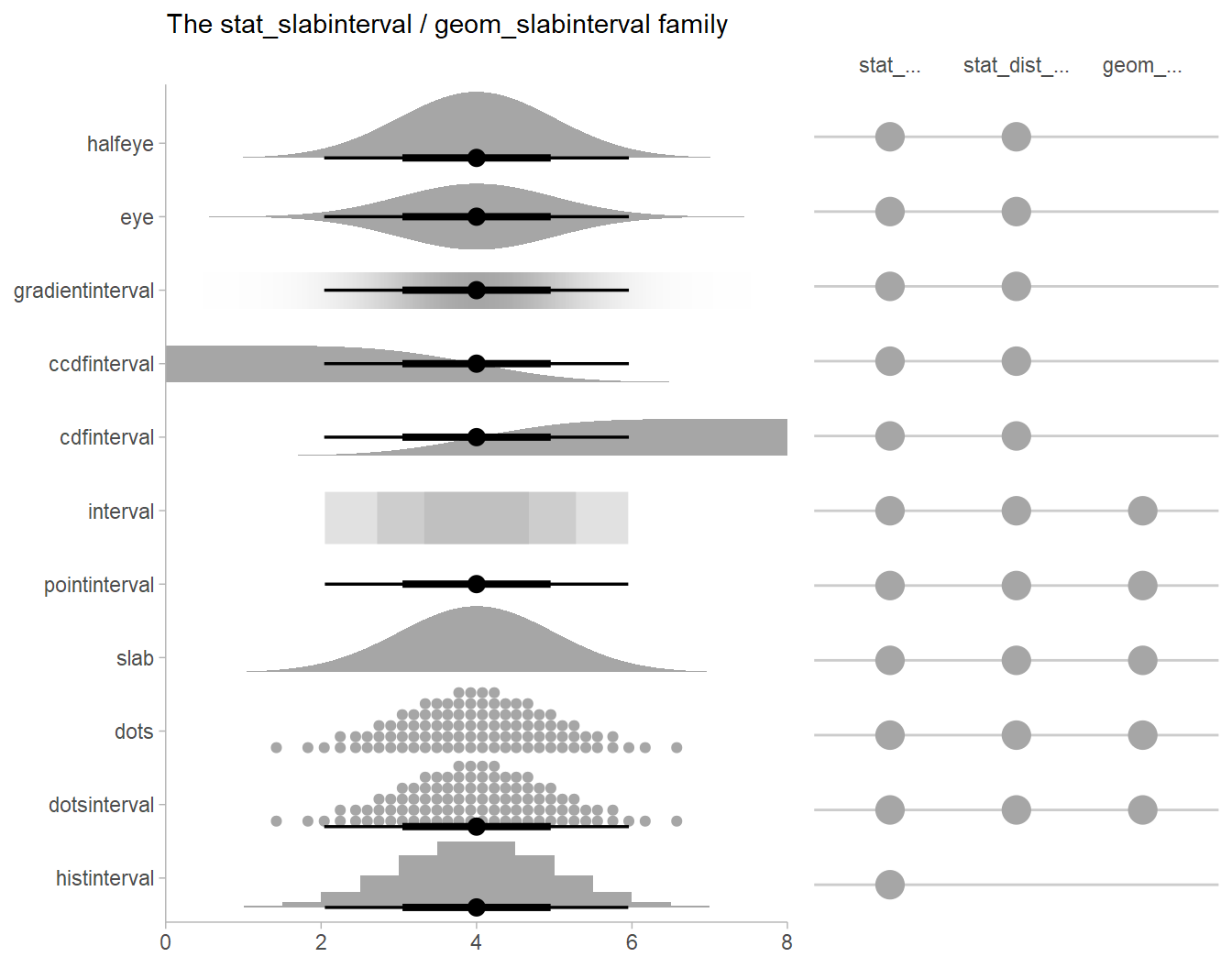See `vignette("slabinterval", package = "ggdist")` for an overview.

## Posterior fits

Rather than calculating conditional means manually as in the previous example, we could use `add_fitted_draws()`, which is analogous to `brms::fitted.brmsfit()` or `brms::posterior_linpred()` (giving posterior draws from the model’s linear predictor, in this case, posterior distributions of conditional means), but uses a tidy data format. We can combine it with `modelr::data_grid()` to first generate a grid describing the fits we want, then transform that grid into a long-format data frame of draws from posterior fits:

``````ABC %>%
data_grid(condition) %>%
condition .row .chain .iteration .draw .value
A 1 NA NA 1 0.1617577
A 1 NA NA 2 0.1051590
A 1 NA NA 3 0.2387882
A 1 NA NA 4 0.3189038
A 1 NA NA 5 0.1303770
A 1 NA NA 6 0.1909871
A 1 NA NA 7 0.2797993
A 1 NA NA 8 -0.0274069
A 1 NA NA 9 0.2608911
A 1 NA NA 10 0.2363987

To plot this example, we’ll also show the use of `ggdist::stat_pointinterval()` instead of `ggdist::geom_pointinterval()`, which summarizes draws into points and intervals within ggplot:

``````ABC %>%
data_grid(condition) %>%
ggplot(aes(x = .value, y = condition)) +
stat_pointinterval(.width = c(.66, .95))``````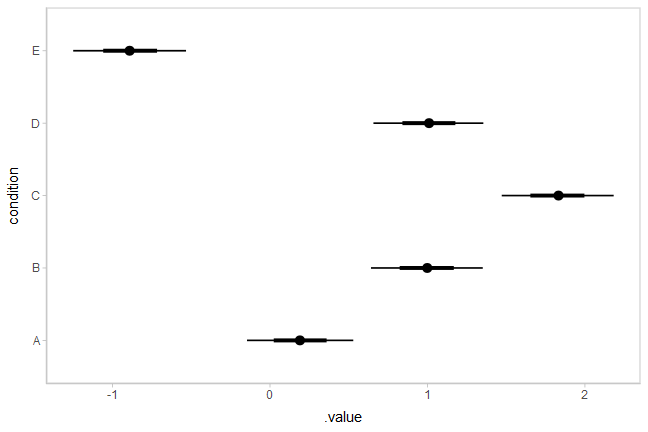## Quantile dotplots

Intervals are nice if the alpha level happens to line up with whatever decision you are trying to make, but getting a shape of the posterior is better (hence eye plots, above). On the other hand, making inferences from density plots is imprecise (estimating the area of one shape as a proportion of another is a hard perceptual task). Reasoning about probability in frequency formats is easier, motivating quantile dotplots (Kay et al. 2016, Fernandes et al. 2018), which also allow precise estimation of arbitrary intervals (down to the dot resolution of the plot, 100 in the example below).

Within the slabinterval family of geoms in tidybayes is the `dots` and `dotsinterval` family, which automatically determine appropriate bin sizes for dotplots and can calculate quantiles from samples to construct quantile dotplots. `ggdist::stat_dots()` is the variant designed for use on samples:

``````ABC %>%
data_grid(condition) %>%
ggplot(aes(x = .value, y = condition)) +
stat_dots(quantiles = 100)``````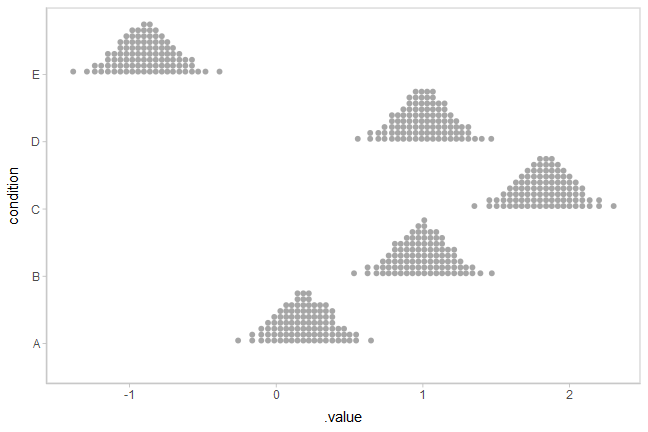The idea is to get away from thinking about the posterior as indicating one canonical point or interval, but instead to represent it as (say) 100 approximately equally likely points.

## Posterior predictions

Where `add_fitted_draws()` is analogous to `brms::fitted.brmsfit()` (or `brms::posterior_linpred()`), `add_predicted_draws()` is analogous to `brms::predict.brmsfit()` (or `brms::posterior_predict()`), giving draws from the posterior predictive distribution.

Here is an example of posterior predictive distributions plotted using `ggdist::stat_slab()`:

``````ABC %>%
data_grid(condition) %>%
ggplot(aes(x = .prediction, y = condition)) +
stat_slab()``````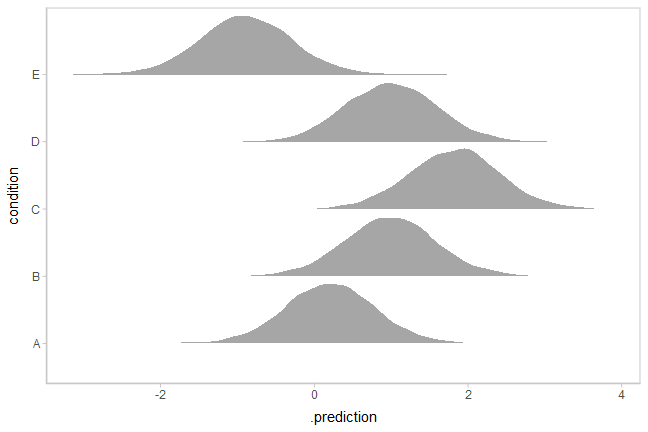We could also use `ggdist::stat_interval()` to plot predictive bands alongside the data:

``````ABC %>%
data_grid(condition) %>%
ggplot(aes(y = condition, x = .prediction)) +
stat_interval(.width = c(.50, .80, .95, .99)) +
geom_point(aes(x = response), data = ABC) +
scale_color_brewer()``````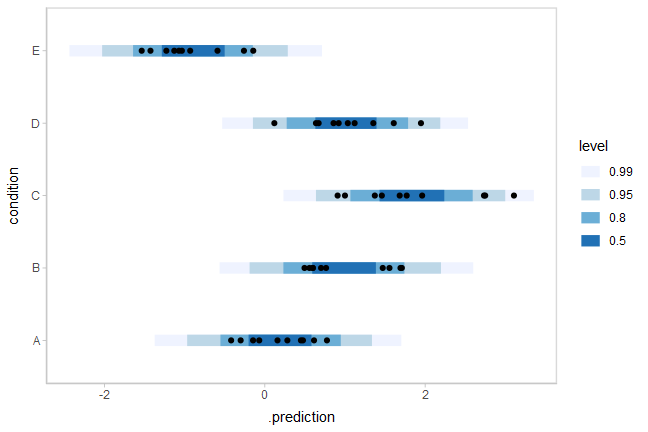Altogether, data, posterior predictions, and posterior distributions of the means:

``````grid = ABC %>%
data_grid(condition)

fits = grid %>%

preds = grid %>%

ABC %>%
ggplot(aes(y = condition, x = response)) +
stat_interval(aes(x = .prediction), data = preds) +
stat_pointinterval(aes(x = .value), data = fits, .width = c(.66, .95), position = position_nudge(y = -0.3)) +
geom_point() +
scale_color_brewer()``````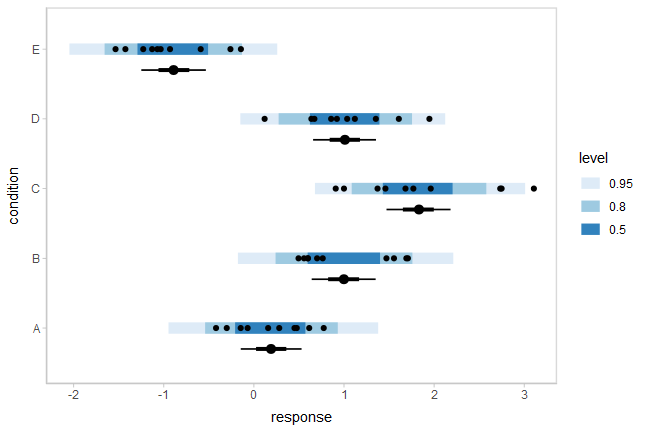## Posterior predictions, Kruschke-style

The above approach to posterior predictions integrates over the parameter uncertainty to give a single posterior predictive distribution. Another approach, often used by John Kruschke in his book Doing Bayesian Data Analysis, is to attempt to show both the predictive uncertainty and the parameter uncertainty simultaneously by showing several possible predictive distributions implied by the posterior.

We can do this pretty easily by asking for the distributional parameters for a given prediction implied by the posterior. We’ll do it explicitly here by setting `dpar = c("mu", "sigma")` in `add_fitted_draws()`. Rather than specifying the parameters explicitly, you can also just set `dpar = TRUE` to get draws from all distributional parameters in a model, and this will work for any response distribution supported by brms. Then, we can select a small number of draws using `sample_draws()` and then use `ggdist::stat_dist_slab()` to visualize each predictive distribution implied by the values of `mu` and `sigma`:

``````ABC %>%
data_grid(condition) %>%
add_fitted_draws(m, dpar = c("mu", "sigma")) %>%
sample_draws(30) %>%
ggplot(aes(y = condition)) +
stat_dist_slab(aes(dist = "norm", arg1 = mu, arg2 = sigma),
slab_color = "gray65", alpha = 1/10, fill = NA
) +
geom_point(aes(x = response), data = ABC, shape = 21, fill = "#9ECAE1", size = 2)``````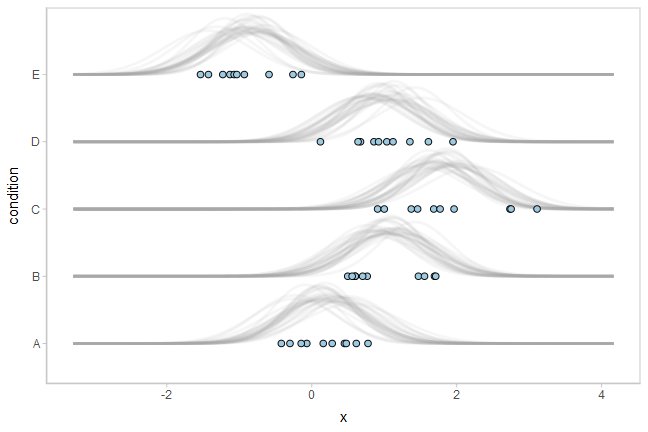For a more detailed description of these charts (and some useful variations on them), see Solomon Kurz’s excellent blog post on the topic.

We could even combine the Kruschke-style plots of predictive distributions with half-eyes showing the posterior means:

``````ABC %>%
data_grid(condition) %>%
add_fitted_draws(m, dpar = c("mu", "sigma")) %>%
ggplot(aes(x = condition)) +
stat_dist_slab(aes(dist = "norm", arg1 = mu, arg2 = sigma),
slab_color = "gray65", alpha = 1/10, fill = NA, data = . %>% sample_draws(30), scale = .5
) +
stat_halfeye(aes(y = .value), side = "bottom", scale = .5) +
geom_point(aes(y = response), data = ABC, shape = 21, fill = "#9ECAE1", size = 2, position = position_nudge(x = -.2))``````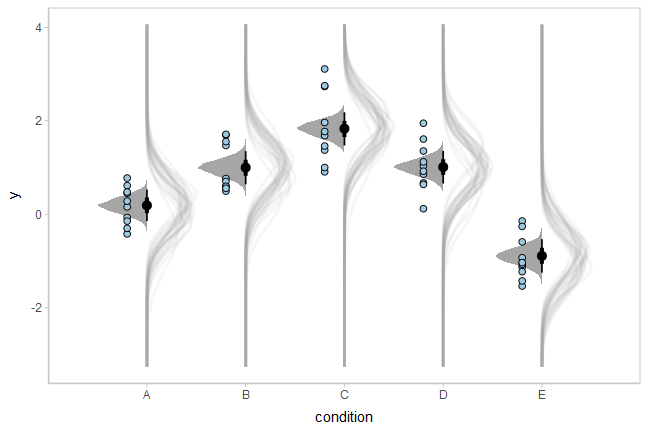## Fit/prediction curves

To demonstrate drawing fit curves with uncertainty, let’s fit a slightly naive model to part of the `mtcars` dataset:

``````m_mpg = brm(
mpg ~ hp * cyl,
data = mtcars,

file = "models/tidy-brms_m_mpg.rds"  # cache model (can be removed)
)``````

We can draw fit curves with probability bands:

``````mtcars %>%
group_by(cyl) %>%
data_grid(hp = seq_range(hp, n = 51)) %>%
ggplot(aes(x = hp, y = mpg, color = ordered(cyl))) +
stat_lineribbon(aes(y = .value)) +
geom_point(data = mtcars) +
scale_fill_brewer(palette = "Greys") +
scale_color_brewer(palette = "Set2")``````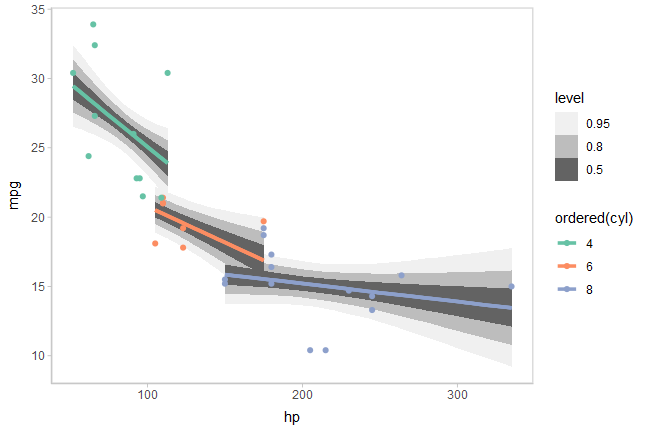Or we can sample a reasonable number of fit lines (say 100) and overplot them:

``````mtcars %>%
group_by(cyl) %>%
data_grid(hp = seq_range(hp, n = 101)) %>%
ggplot(aes(x = hp, y = mpg, color = ordered(cyl))) +
geom_line(aes(y = .value, group = paste(cyl, .draw)), alpha = .1) +
geom_point(data = mtcars) +
scale_color_brewer(palette = "Dark2")``````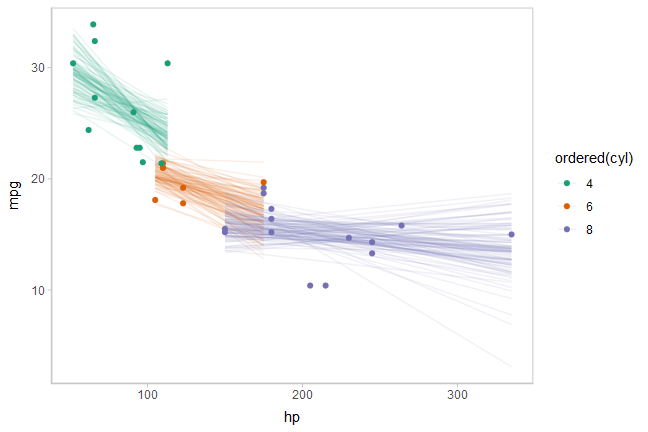Or we can create animated hypothetical outcome plots (HOPs) of fit lines:

``````set.seed(123456)
ndraws = 50

p = mtcars %>%
group_by(cyl) %>%
data_grid(hp = seq_range(hp, n = 101)) %>%
ggplot(aes(x = hp, y = mpg, color = ordered(cyl))) +
geom_line(aes(y = .value, group = paste(cyl, .draw))) +
geom_point(data = mtcars) +
scale_color_brewer(palette = "Dark2") +
transition_states(.draw, 0, 1) +
shadow_mark(future = TRUE, color = "gray50", alpha = 1/20)

animate(p, nframes = ndraws, fps = 2.5, width = 432, height = 288, res = 96, dev = "png", type = "cairo")``````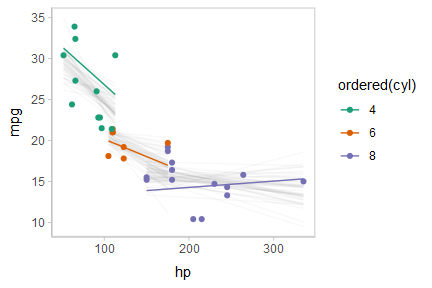Or, for posterior predictions (instead of fits), we can go back to probability bands:

``````mtcars %>%
group_by(cyl) %>%
data_grid(hp = seq_range(hp, n = 101)) %>%
ggplot(aes(x = hp, y = mpg, color = ordered(cyl), fill = ordered(cyl))) +
stat_lineribbon(aes(y = .prediction), .width = c(.95, .80, .50), alpha = 1/4) +
geom_point(data = mtcars) +
scale_fill_brewer(palette = "Set2") +
scale_color_brewer(palette = "Dark2")``````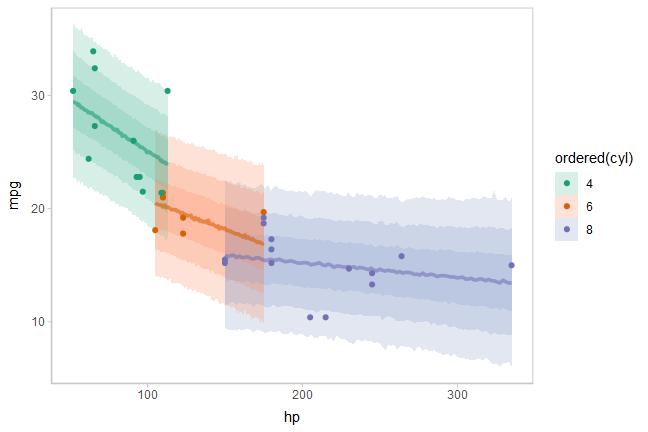This gets difficult to judge by group, so probably better to facet into multiple plots. Fortunately, since we are using ggplot, that functionality is built in:

``````mtcars %>%
group_by(cyl) %>%
data_grid(hp = seq_range(hp, n = 101)) %>%
ggplot(aes(x = hp, y = mpg)) +
stat_lineribbon(aes(y = .prediction), .width = c(.99, .95, .8, .5), color = brewer.pal(5, "Blues")[]) +
geom_point(data = mtcars) +
scale_fill_brewer() +
facet_grid(. ~ cyl, space = "free_x", scales = "free_x")``````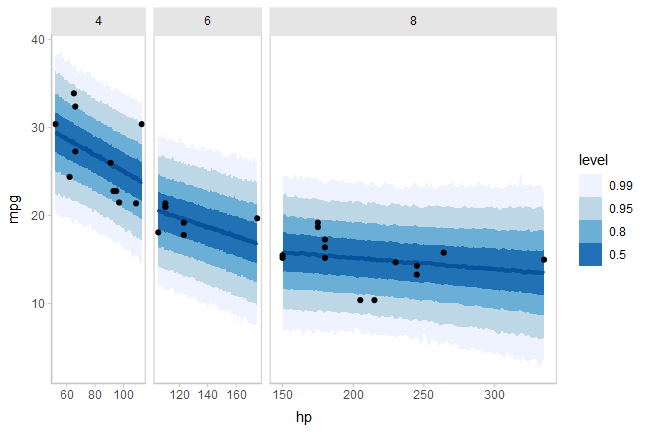### Extracting distributional regression parameters

`brms::brm()` also allows us to set up submodels for parameters of the response distribution other than the location (e.g., mean). For example, we can allow a variance parameter, such as the standard deviation, to also be some function of the predictors.

This approach can be helpful in cases of non-constant variance (also called heteroskedasticity by folks who like obfuscation via Latin). E.g., imagine two groups, each with different mean response and variance:

``````set.seed(1234)
AB = tibble(
group = rep(c("a", "b"), each = 20),
response = rnorm(40, mean = rep(c(1, 5), each = 20), sd = rep(c(1, 3), each = 20))
)

AB %>%
ggplot(aes(x = response, y = group)) +
geom_point()``````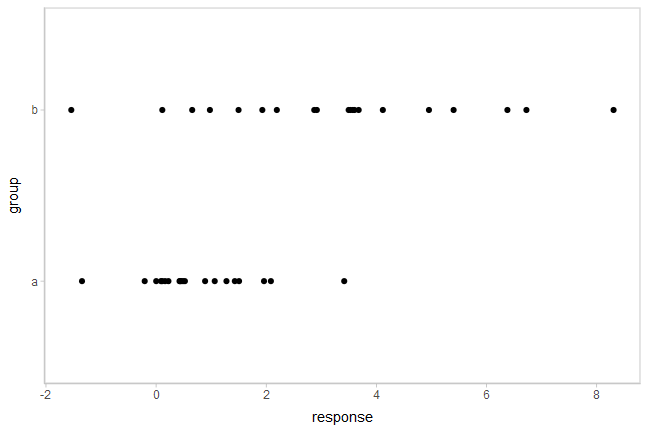Here is a model that lets the mean and standard deviation of `response` be dependent on `group`:

``````m_ab = brm(
bf(
response ~ group,
sigma ~ group
),
data = AB,

file = "models/tidy-brms_m_ab.rds"  # cache model (can be removed)
)``````

We can plot the posterior distribution of the mean `response` alongside posterior predictive intervals and the data:

``````grid = AB %>%
data_grid(group)

fits = grid %>%

preds = grid %>%

AB %>%
ggplot(aes(x = response, y = group)) +
stat_halfeye(aes(x = .value), scale = 0.6, position = position_nudge(y = 0.175), data = fits) +
stat_interval(aes(x = .prediction), data = preds) +
geom_point(data = AB) +
scale_color_brewer()``````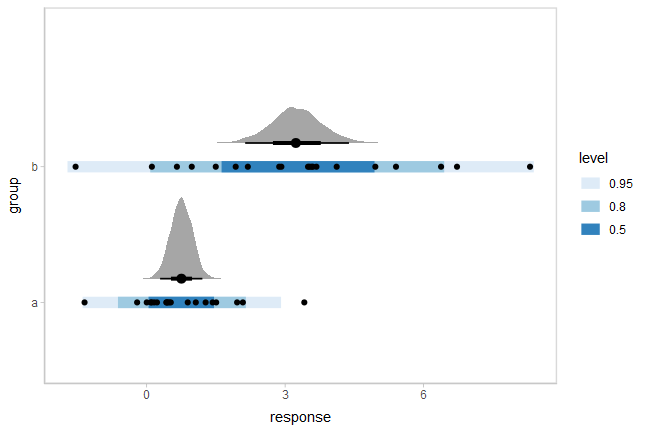This shows posteriors of the mean of each group (black intervals and the density plots) and posterior predictive intervals (blue).

The predictive intervals in group `b` are larger than in group `a` because the model fits a different standard deviation for each group. We can see how the corresponding distributional parameter, `sigma`, changes by extracting it using the `dpar` argument to `add_fitted_draws()`:

``````grid %>%
ggplot(aes(x = sigma, y = group)) +
stat_halfeye() +
geom_vline(xintercept = 0, linetype = "dashed")``````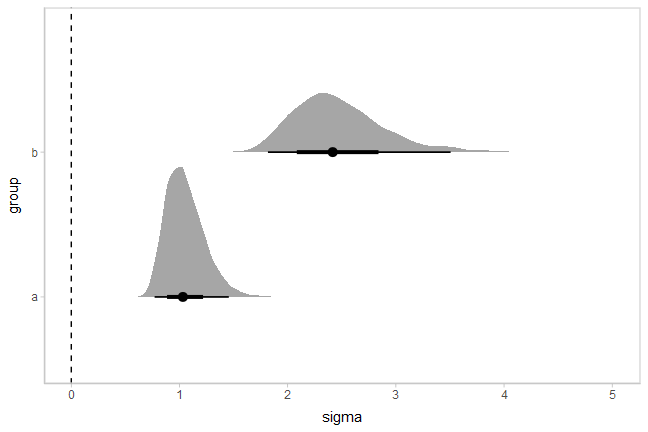By setting `dpar = TRUE`, all distributional parameters are added as additional columns in the result of `add_fitted_draws()`; if you only want a specific parameter, you can specify it (or a list of just the parameters you want). In the above model, `dpar = TRUE` is equivalent to `dpar = list("mu", "sigma")`.

## Comparing levels of a factor

If we wish compare the means from each condition, `compare_levels()` facilitates comparisons of the value of some variable across levels of a factor. By default it computes all pairwise differences.

Let’s demonstrate `compare_levels()` with `ggdist::stat_halfeye()`:

``````#N.B. the syntax for compare_levels is experimental and may change
m %>%
compare_levels(r_condition, by = condition) %>%
ggplot(aes(y = condition, x = r_condition)) +
stat_halfeye()``````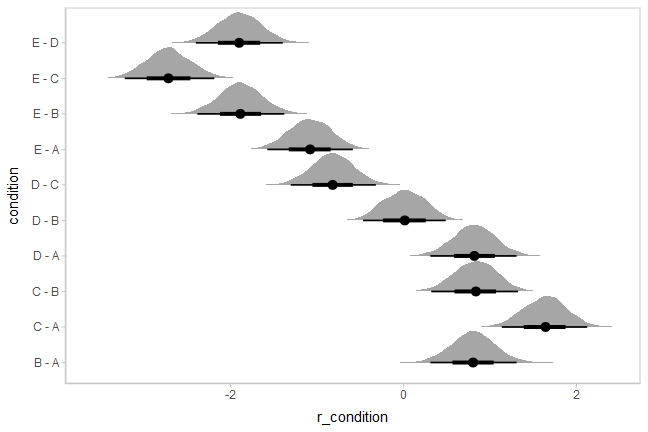If you prefer “caterpillar” plots, ordered by something like the mean of the difference, you can reorder the factor before plotting:

``````#N.B. the syntax for compare_levels is experimental and may change
m %>%
compare_levels(r_condition, by = condition) %>%
ungroup() %>%
mutate(condition = reorder(condition, r_condition)) %>%
ggplot(aes(y = condition, x = r_condition)) +
stat_halfeye() +
geom_vline(xintercept = 0, linetype = "dashed") ``````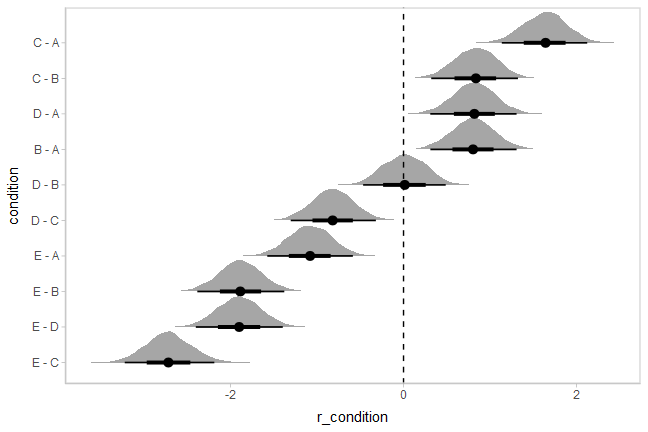## Ordinal models

The `brms::fitted.brmsfit()` function for ordinal and multinomial regression models in brms returns multiple variables for each draw: one for each outcome category (in contrast to `rstanarm::stan_polr()` models, which return draws from the latent linear predictor). The philosophy of `tidybayes` is to tidy whatever format is output by a model, so in keeping with that philosophy, when applied to ordinal and multinomial `brms` models, `add_fitted_draws()` adds an additional column called `.category` and a separate row containing the variable for each category is output for every draw and predictor.

### Ordinal model with continuous predictor

We’ll fit a model using the `mtcars` dataset that predicts the number of cylinders in a car given the car’s mileage (in miles per gallon). While this is a little backwards causality-wise (presumably the number of cylinders causes the mileage, if anything), that does not mean this is not a fine prediction task (I could probably tell someone who knows something about cars the MPG of a car and they could do reasonably well at guessing the number of cylinders in the engine).

Before we fit the model, let’s clean the dataset by making the `cyl` column an ordered factor (by default it is just a number):

``````mtcars_clean = mtcars %>%
mutate(cyl = ordered(cyl))

mpg cyl disp hp drat wt qsec vs am gear carb
21.0 6 160 110 3.90 2.620 16.46 0 1 4 4
21.0 6 160 110 3.90 2.875 17.02 0 1 4 4
22.8 4 108 93 3.85 2.320 18.61 1 1 4 1
21.4 6 258 110 3.08 3.215 19.44 1 0 3 1
18.7 8 360 175 3.15 3.440 17.02 0 0 3 2
18.1 6 225 105 2.76 3.460 20.22 1 0 3 1

Then we’ll fit an ordinal regression model:

``````m_cyl = brm(
cyl ~ mpg,
data = mtcars_clean,
family = cumulative,
seed = 58393,

file = "models/tidy-brms_m_cyl.rds"  # cache model (can be removed)
)``````

`add_fitted_draws()` will include a `.category` column, and `.value` will contain draws from the posterior distribution for the probability that the response is in that category. For example, here is the fit for the first row in the dataset:

``````tibble(mpg = 21) %>%
median_qi(.value)``````
mpg .row .category .value .lower .upper .width .point .interval
21 1 1 0.3450535 0.0980623 0.7023782 0.95 median qi
21 1 2 0.6268533 0.2783143 0.8899112 0.95 median qi
21 1 3 0.0133046 0.0002549 0.1184035 0.95 median qi

Note how the `.category` variable does not retain the original factor level names. This is due to a bug in brms 2.11 (see here). As a workaround, we can recover the original factor labels and assign the result to a `cyl` column:

``````tibble(mpg = 21) %>%

# recover original factor labels
mutate(cyl = levels(mtcars_clean\$cyl)[.category]) %>%

median_qi(.value)``````
mpg .row .category cyl .value .lower .upper .width .point .interval
21 1 1 4 0.3450535 0.0980623 0.7023782 0.95 median qi
21 1 2 6 0.6268533 0.2783143 0.8899112 0.95 median qi
21 1 3 8 0.0133046 0.0002549 0.1184035 0.95 median qi

We could plot fit lines for fitted probabilities against the dataset:

``````data_plot = mtcars_clean %>%
ggplot(aes(x = mpg, y = cyl, color = cyl)) +
geom_point() +
scale_color_brewer(palette = "Dark2", name = "cyl")

fit_plot = mtcars_clean %>%
data_grid(mpg = seq_range(mpg, n = 101)) %>%
add_fitted_draws(m_cyl, value = "P(cyl | mpg)") %>%
# recover original factor labels
mutate(cyl = levels(mtcars_clean\$cyl)[.category]) %>%
ggplot(aes(x = mpg, y = `P(cyl | mpg)`, color = cyl)) +
stat_lineribbon(aes(fill = cyl), alpha = 1/5) +
scale_color_brewer(palette = "Dark2") +
scale_fill_brewer(palette = "Dark2")

plot_grid(ncol = 1, align = "v",
data_plot,
fit_plot
)``````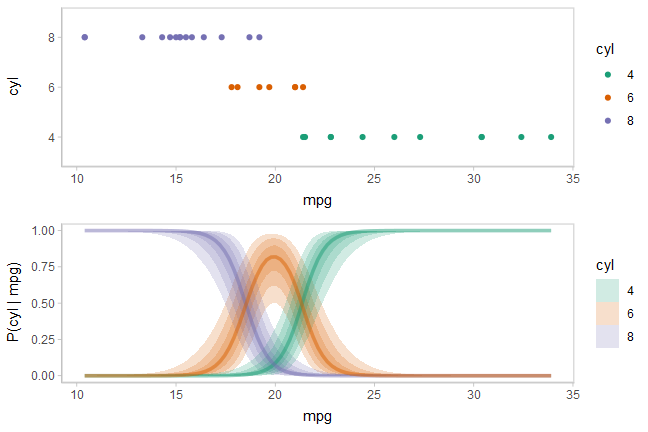The above display does not let you see the correlation between `P(cyl|mpg)` for different values of `cyl` at a particular value of `mpg`. For example, in the portion of the posterior where `P(cyl = 6|mpg = 20)` is high, `P(cyl = 4|mpg = 20)` and `P(cyl = 8|mpg = 20)` must be low (since these must add up to 1).

One way to see this correlation might be to employ hypothetical outcome plots (HOPs) just for the fit line, “detaching” it from the ribbon (another alternative would be to use HOPs on top of line ensembles, as demonstrated earlier in this document). By employing animation, you can see how the lines move in tandem or opposition to each other, revealing some patterns in how they are correlated:

``````ndraws = 50

p = mtcars_clean %>%
data_grid(mpg = seq_range(mpg, n = 101)) %>%
add_fitted_draws(m_cyl, value = "P(cyl | mpg)") %>%
# recover original factor labels
mutate(cyl = levels(mtcars_clean\$cyl)[.category]) %>%
ggplot(aes(x = mpg, y = `P(cyl | mpg)`, color = cyl)) +
# we remove the `.draw` column from the data for stat_lineribbon so that the same ribbons
# are drawn on every frame (since we use .draw to determine the transitions below)
stat_lineribbon(aes(fill = cyl), alpha = 1/5, color = NA, data = . %>% select(-.draw)) +
# we use sample_draws to subsample at the level of geom_line (rather than for the full dataset
# as in previous HOPs examples) because we need the full set of draws for stat_lineribbon above
geom_line(aes(group = paste(.draw, cyl)), size = 1, data = . %>% sample_draws(ndraws)) +
scale_color_brewer(palette = "Dark2") +
scale_fill_brewer(palette = "Dark2") +
transition_manual(.draw)

animate(p, nframes = ndraws, fps = 2.5, width = 576, height = 192, res = 96, dev = "png", type = "cairo")``````